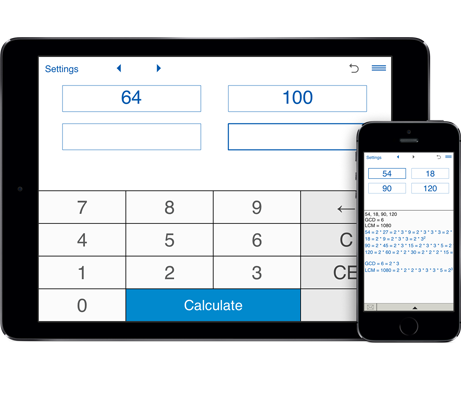# GCD and LCM calculator

Finds the greatest common divisor (GCD) and Least Common Multiple (LCM) of two, three and four numbers.
Finds the prime factorization of numbers and shows it in the standard and exponential forms.

## Greatest Common Divisor Calculator and Least Common Multiple Calculator

This is a handy calculator that helps you find the Greatest Common Divisor (GCD) and Least Common Multiple (LCM) of two, three and four numbers and list the prime factors of each number.

Greatest Common Divisor (GCD), also known as Greatest Common Factor (GCF) or Highest Common Factor (HCF), of two or more positive integers is the largest positive integer that divides all these numbers without a remainder.

Least Common Multiple (LCM) also called the Lowest Common Multiple or Smallest Common Multiple of two or more integers is the smallest number that is a multiple of the numbers.

The GCD calculator and LCM calculator will find the greatest common divisor (GCD) and least common multiple (LCM) of two, three and four integers.

#### How to find the GCD and LCM

To find the Greatest Common Divisor and Lowest Common Multiple, simply enter the numbers and click Calculate.
The GCD calculator and LCM calculator will calculate the Greatest Common Divisor (GCD) and the Least Common Multiple (LCM) of numbers and their prime factors.